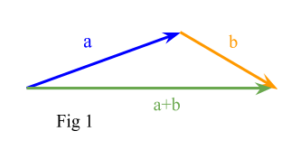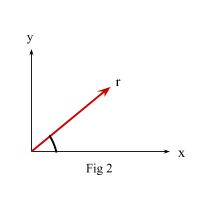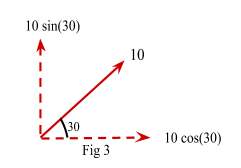# Vectors

Contents

## Summary

• A unit vector$\widehat { r }$  is the vector whose magnitude is 1 unit. It is used to specify the direction of the given vector.
• Unit vector is defined as:$\widehat { r } \quad =\quad \frac { ai\quad +\quad bj\quad +\quad ck }{ \sqrt { { (a) }^{ 2 }\quad +\quad { (b) }^{ 2 }\quad +\quad { (c) }^{ 2 } } }$
• Magnitude of a vector is:$\left| r \right| \quad =\quad \sqrt { { (a) }^{ 2 }\quad +\quad { (b) }^{ 2 }\quad +\quad { (c) }^{ 2 } }$
• A vector can be further broken down or resolved into its two components: vertical and horizontal. Vertical component is defined as${ r }_{ y }\quad =\quad r\quad sin\theta$  and horizontal component is defined as${ r }_{ x }\quad =\quad r\quad cos\theta$ .

Vector quantities are extremely useful in physics. The important characteristic of a vector quantity is that it has both a magnitude and a direction. Both of these properties must be given in order to specify a vector completely.

#### Unit vector

A unit vector$\widehat { r }$  is the vector whose magnitude is 1 unit. It is used to specify the direction of the given vector. Also:$\widehat { r } \quad =\quad \frac { r }{ \left| r \right| }$   i.e

If a vector is divided by its magnitude (modulus) then we get a unit vector in the direction of that vector.

Unit vectors can be described as i + j+ k, where i is the direction of the x axis and j is the direction of the y axis and k is the direction of the z axis.

If r = ai +bj+ck, then the unit vector in the direction of r is given by:$\widehat { r } \quad =\quad \frac { ai\quad +\quad bj\quad +\quad ck }{ \sqrt { { (a) }^{ 2 }\quad +\quad { (b) }^{ 2 }\quad +\quad { (c) }^{ 2 } } }$

The denominator of the above formula is basically the magnitude of the vector, therefore to work out the magnitude of this vector, we use the formula:$\left| r \right| \quad =\quad \sqrt { { (a) }^{ 2 }\quad +\quad { (b) }^{ 2 }\quad +\quad { (c) }^{ 2 } }$

Given two vectors a and b, we form their sum a + b, as follows.

We translate the vector b until its tail coincides with the head of a.

Then, the directed line segment from the tail of a to the head of b is the vector a+b just as shown in Fig 1.#### Example #1

Q. Find the sum of vectors p = 2i + 5j +3k and q = i – 2j + 2k.

Solution:

We carry out the addition of two vectors in the following way:

p + q = (2 + 1)i + (5 – 2)j + (3 + 2)k

p + q = 3i + 3j +5k

#### Example #2

Q. Work out a – b when a = 10i + 12j – 7k and b = 5i – 2j + 8k

Solution:

a – b = (10 – 5)i + (12 – (-2))j + (-7 – 8)k

a – b = 5i+ 10j- 15k

#### Resolving a Vector

A vector can be further broken down or resolved into its two components; vertical and horizontal.

Let’s take an example of a vector in figure 2 (Fig 2)This vector can be resolved into two components:

1.    Vertical component which is along the y axis.
2.    Horizontal component which is along the x axis

We can calculate the vertical and horizontal components if we know the magnitude and direction of the vector.

In other words: we can work out the components of the vector if we know the length of the line and the angle between it and the horizontal or vertical axis.

Let’s suppose vector r in Fig 2, its vertical component is defined as${ r }_{ y }\quad =\quad r\quad sin\theta$,  and its horizontal component is defined as${ r }_{ x }\quad =\quad r\quad cos\theta$.

Therefore:$r\quad =\quad (r\quad sin\theta )i\quad +\quad (r\quad cos\theta )j$

#### Example #3

Q. Find vector r given that magnitude of$\left| r \right| \quad =\quad 10$  and angle between the vector and its horizontal component is 30°.

Solution:

We resolve the vector into two components as shown in Fig 3 below.Therefore:

• vertical component:${ r }_{ y }\quad =\quad 10\quad sin(30)$${ r }_{ y }\quad =\quad 5$

• horizontal component:${ r }_{ x }\quad =\quad 10\quad cos(30)$${ r }_{ x }\quad =\quad 8.66$

Hence:$r\quad =\quad { r }_{ x }i\quad +\quad { r }_{ y }j$

r = 5i + 8.66 j